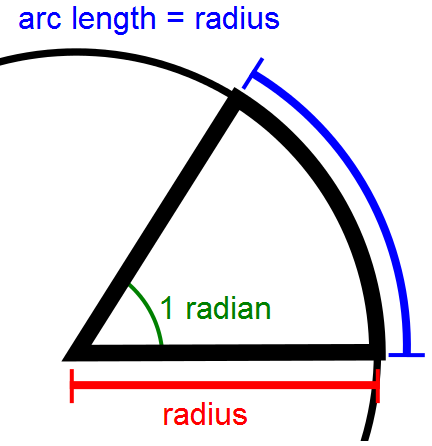### Introduction

In physics, the angular velocity is a vector quantity (more precisely, a pseudo-vector) which specifies the angular speed of an object and the axis about which the object is rotating. The Unit (Metric System) of angular velocity is radians per second,  although it may be measured in other units such as degrees per second, revolutions per second, revolutions per minute, degrees per hour, etc. It is sometimes also called the rotational velocity and its magnitude the rotational speed, typically measured in cycles or rotations per unit time (e.g. revolutions per minute). Angular velocity is usually represented by the symbol omega (ω, rarely Ω).The radian per second is defined as the change in the orientation of an object, in radians, every second.

Angular frequency ω(Ordinary) frequency ν = ω / 2π
2π radians per secondexactly 1 hertz (Hz)
1 radian per secondapproximately 0.159155 Hz
1 radian per secondapproximately 57.29578 degrees per second
1 radian per secondapproximately 9.5493 revolutions per minute